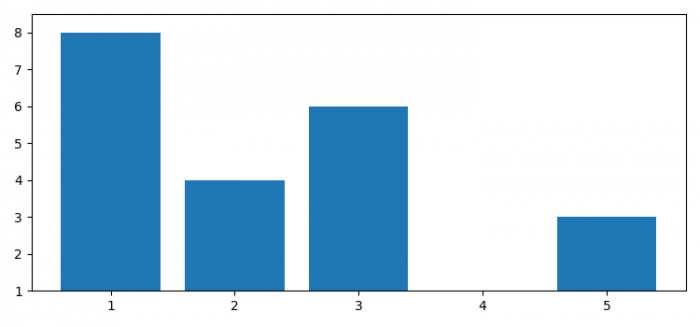# Automatically setting Y-axis limits for a bar graph using Matplotlib

MatplotlibPythonData Visualization

To set Y-axis limit, we can use ylim() method and put maximum and minimum limit values.

## Steps

• Set the figure size and adjust the padding between and around the subplots.
• Create two lists for data points.
• Make two variables for max and min values for Y-axis.
• Use ylim() method to limit the Y-axis range.
• Use bar() method to plot the bars.
• To display the figure, use show() method.

## Example

from matplotlib import pyplot as plt

plt.rcParams["figure.figsize"] = [7.50, 3.50]
plt.rcParams["figure.autolayout"] = True
x = [1, 2, 3, 4, 5]
y = [8, 4, 6, 1, 3]
max_y_lim = max(y) + .5
min_y_lim = min(y)
plt.ylim(min_y_lim, max_y_lim)
plt.bar(x, y)
plt.show()

## Output